Help: FAQs, overviews and step by step guides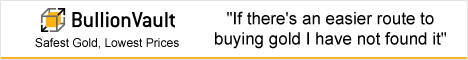BV_468x60_v1.gif: width=468, height=60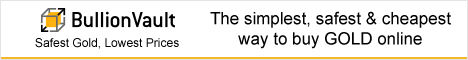BV_468x60_v2.gif: width=468, height=60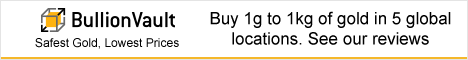BV_468x60_v3.gif: width=468, height=60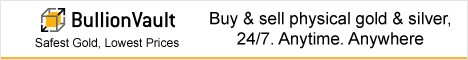BV_468x60_v4.gif: width=468, height=60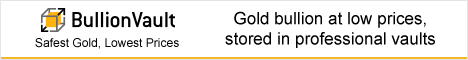BV_468x60_v5.gif: width=468, height=60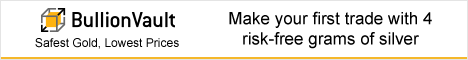BV_468x60_v6.gif: width=468, height=60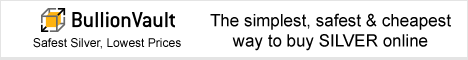BV_468x60_v7.gif: width=468, height=60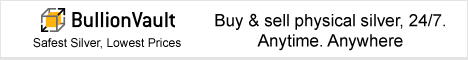BV_468x60_v8.gif: width=468, height=60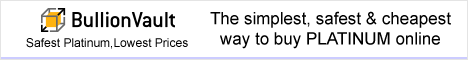BV_468x60_v9.gif: width=468, height=60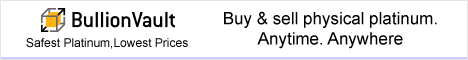BV_468x60_v10.gif: width=468, height=60BV_728x90_v1.gif: width=728, height=90BV_728x90_v2.gif: width=728, height=90BV_728x90_v3.gif: width=728, height=90BV_728x90_v4.gif: width=728, height=90BV_728x90_v5.gif: width=728, height=90BV_728x90_v6.gif: width=728, height=90BV_728x90_v7.gif: width=728, height=90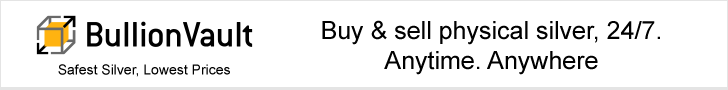BV_728x90_v8.gif: width=728, height=90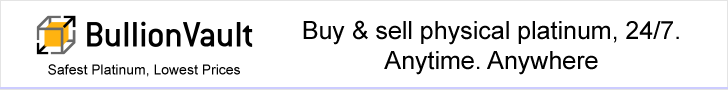BV_728x90_v9.gif: width=728, height=90BV_728x90_v10.gif: width=728, height=90BV_300x250_v1.gif: width=300, height=250BV_300x250_v2.gif: width=300, height=250BV_300x250_v3.gif: width=300, height=250BV_300x250_v4.gif: width=300, height=250BV_300x250_v5.gif: width=300, height=250BV_300x250_v6.gif: width=300, height=250BV_300x250_v7.gif: width=300, height=250BV_300x250_v8.gif: width=300, height=250BV_300x250_v9.gif: width=300, height=250BV_300x250_v10.gif: width=300, height=250BV_120x600_v1.gif: width=120, height=600BV_120x600_v2.gif: width=120, height=600BV_120x600_v3.gif: width=120, height=600BV_120x600_v4.gif: width=120, height=600BV_120x600_v5.gif: width=120, height=600BV_120x600_v6.gif: width=120, height=600BV_120x600_v7.gif: width=120, height=600BV_120x600_v8.gif: width=120, height=600BV_120x600_v9.gif: width=120, height=600BV_160x600_v1.gif: width=160, height=600BV_160x600_v2.gif: width=160, height=600BV_160x600_v3.gif: width=160, height=600BV_160x600_v4.gif: width=160, height=600BV_160x600_v5.gif: width=160, height=600BV_160x600_v6.gif: width=160, height=600BV_160x600_v7.gif: width=160, height=600BV_160x600_v8.gif: width=160, height=600